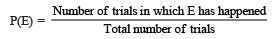Courses

# Facts that Matter- Probability Class 9 Notes | EduRev

## Mathematics (Maths) Class 9

Created by: Full Circle

## Class 9 : Facts that Matter- Probability Class 9 Notes | EduRev

The document Facts that Matter- Probability Class 9 Notes | EduRev is a part of the Class 9 Course Mathematics (Maths) Class 9.
All you need of Class 9 at this link: Class 9

Facts that Matter

• An operation which can produce some well-defined outcomes is called an experiment.
• An experiment in which all possible outcomes are known and the exact outcome cannot be predicted in advance, is called a random experiment.
• Performing a random experiment is called trial.
• The empirical (or experimental) probability P(E) of an event E is given by• The probability of an event lies between 0 and 1.

UNCERTAINTY
A doubtful situation of an action or activity that may or may not take place, is called ‘uncertainty’.

PROBABILITY
The numerical measure of uncertainty of an action (or activity) is called probability.

Note:
The words ‘doubt’, ‘chances’, ‘most probably’ etc., show uncertainty or probability of happening of an action or activity.

,

,

,

,

,

,

,

,

,

,

,

,

,

,

,

,

,

,

,

,

,

;